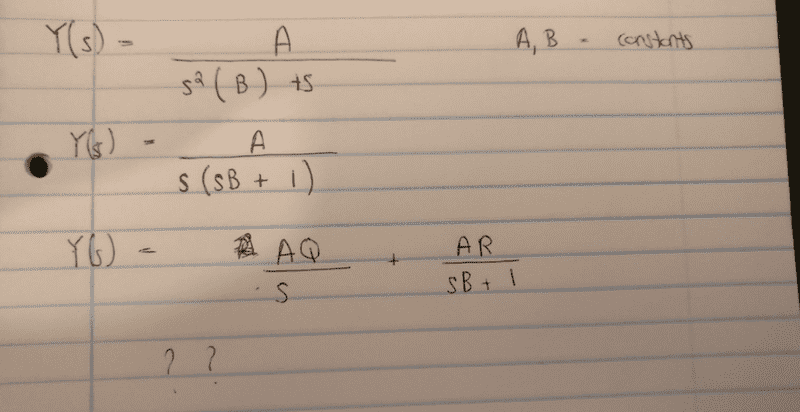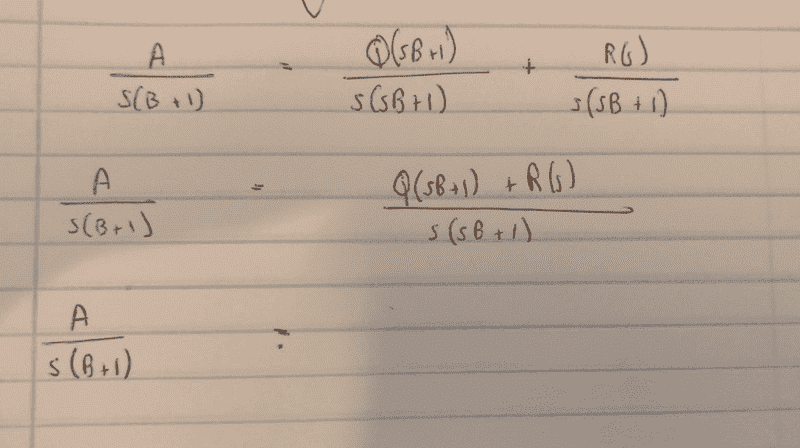# Solving ODEs with Laplace. Stuck at Partial Fraction Expansi

CoolDude420

## Homework Statement

Hi,
So I had a pretty long question solving a Linear ODE but now I've gotten stuck at this stage where I can't seem to get it into the right form to carry out partial fraction expansion

## The Attempt at a Solution[/B]
I'm quite sure that I what I have at the very last line isn't correct. I'm really new to solving ODEs with Laplace. Q and R are the constant things that you put over the fraction when solving with partial fraction expansion.

Homework Helper
Gold Member

## Homework Statement

Hi,
So I had a pretty long question solving a Linear ODE but now I've gotten stuck at this stage where I can't seem to get it into the right form to carry out partial fraction expansion

## The Attempt at a Solution

View attachment 213056
[/B]
I'm quite sure that I what I have at the very last line isn't correct. I'm really new to solving ODEs with Laplace. Q and R are the constant things that you put over the fraction when solving with partial fraction expansion.
You have$$Y(s)=\frac{A}{s(sB+1)}$$You want to set that equal to its partial fractions like this:$$\frac{A}{s(sB+1)} = \frac Q s + \frac{R}{sB+1}$$
Add the two fractions on the right and compare numerators with the left to get ##Q## and ##R##.

CoolDude420
You have$$Y(s)=\frac{A}{s(sB+1)}$$You want to set that equal to its partial fractions like this:$$\frac{A}{s(sB+1)} = \frac Q s + \frac{R}{sB+1}$$
Add the two fractions on the right and compare numerators with the left to get ##Q## and ##R##.Not sure how to compare these?